### 5.5.4 Modifying shapes

#### Modifying ties and slurs

`Tie`s, `Slur`s, `PhrasingSlur`s, `LaissezVibrerTie`s and `RepeatTie`s are all drawn as third-order Bézier curves. If the shape of the tie or slur which is calculated automatically is not optimum, the shape may be modified manually in two ways:

1. by specifying the displacements to be made to the control points of the automatically calculated Bézier curve, or
2. by explicitly specifying the positions of the four control points required to define the wanted curve.

Both methods are explained below. The first method is more suitable if only slight adjustments to the curve are required; the second may be better for creating curves which are related to just a single note.

#### Cubic Bézier curves

Third-order or cubic Bézier curves are defined by four control points. The first and fourth control points are precisely the starting and ending points of the curve. The intermediate two control points define the shape. Animations showing how the curve is drawn can be found on the web, but the following description and image may be helpful. The curve starts from the first control point heading directly towards the second, gradually bending over to head towards the third and continuing to bend over to head towards the fourth, arriving there travelling directly from the third control point. The curve is entirely contained in the quadrilateral defined by the four control points.Translations, rotations and scaling of the control points all result in exactly the same operations on the curve.

#### Specifying displacements from current control points

In this example the automatic placement of the tie is not optimum, and `\tieDown` would not help.

```<<
{ e'1~ 1 }
\\
\relative { r4 <g' c,> <g c,> <g c,> }
>>
```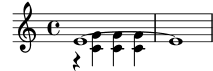Adjusting the control points of the tie with `\shape` allows the collisions to be avoided.

The syntax of `\shape` is

```[-]\shape displacements item
```

This will reposition the control-points of item by the amounts given by displacements. The displacements argument is a list of number pairs or a list of such lists. Each element of a pair represents the displacement of one of the coordinates of a control-point. If item is a string, the result is `\once\override` for the specified grob type. If item is a music expression, the result is the same music expression with an appropriate tweak applied.

In other words, the `\shape` function can act as either a `\once\override` command or a `\tweak` command depending on whether the item argument is a grob name, like “Slur”, or a music expression, like “(”. The displacements argument specifies the displacements of the four control points as a list of four pairs of (dx . dy) values in units of staff-spaces (or a list of such lists if the curve has more than one segment).

The leading hyphen is required if and only if the `\tweak` form is being used.

So, using the same example as above and the `\once\override` form of `\shape`, this will raise the tie by half a staff-space:

```<<
{
\shape #'((0 . 0.5) (0 . 0.5) (0 . 0.5) (0 . 0.5)) Tie
e'1~ 1
}
\\
\relative { r4 <g' c,> <g c,> <g c,> }
>>
```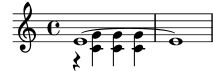This positioning of the tie is better, but maybe it should be raised more in the center. The following example does this, this time using the alternative `\tweak` form:

```<<
{
e'1-\shape #'((0 . 0.5) (0 . 1) (0 . 1) (0 . 0.5)) ~ e'
}
\\
\relative { r4 <g' c,> <g c,> <g c,> }
>>
```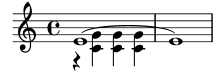To aid the tweaking process, the `\vshape` function is provided. Its name means visual shape: it acts exactly like `\shape`, except that the control points and polygon are additionally displayed.

```\relative {
c''8(\( a) e4 gis a\)
\vshape #'((0 . -0.3) (0.5 . -0.2)
(0.5 . -0.3) (0 . -0.7)) PhrasingSlur
c8(\( a) e4 gis a\)
}
```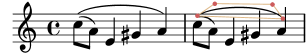It is advisable to start with `\vshape` and adjust until a satisfactory curve is obtained, then simply remove the “v” letter in `\vshape`.

Two different curves starting at the same musical moment may also be shaped:

```\relative {
c''8(\( a) a'4 e c\)
\shape #'((0.7 . -0.4) (0.5 . -0.4)
(0.3 . -0.3) (0 . -0.2)) Slur
\shape #'((0 . 0) (0 . 0.5)
(0 . 0.5) (0 . 0)) PhrasingSlur
c8(\( a) a'4 e c\)
}
```The `\shape` function can also displace the control points of curves which stretch across line breaks. Each piece of the broken curve can be given its own list of offsets. If changes to a particular segment are not needed, the empty list can serve as a placeholder. In this example the line break makes the single slur look like two:

```\relative {
c'4( f g c
\break
d,4 c' f, c)
}
```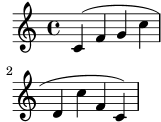Changing the shapes of the two halves of the slur makes it clearer that the slur continues over the line break:

```% () may be used as a shorthand for ((0 . 0) (0 . 0) (0 . 0) (0 . 0))
% if any of the segments does not need to be changed
\relative c' {
\shape #'(
(( 0 . 0) (0 . 0) (0 . 0) (0 . 1))
((0.5 . 1.5) (1 . 0) (0 . 0) (0 . -1.5))
) Slur
c4( f g c
\break
d,4 c' f, c)
}
```If an S-shaped curve is required the control points must always be adjusted manually — LilyPond will never select such shapes automatically.

```\relative c'' {
c8( e b-> f d' a e-> g)
\shape #'((0 . -1) (5.5 . -0.5) (-5.5 . -10.5) (0 . -5.5))
PhrasingSlur
c8\( e b-> f d' a e-> g\)
}
```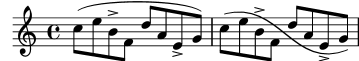#### Specifying control points explicitly

The coordinates of the Bézier control points are specified in units of staff-spaces. The X coordinate is relative to the reference point of the note to which the tie or slur is attached, and the Y coordinate is relative to the staff center line. The coordinates are specified as a list of four pairs of decimal numbers (reals). One approach is to estimate the coordinates of the two end points, and then guess the two intermediate points. The optimum values are then found by trial and error. Be aware that these values may need to be manually adjusted if any further changes are made to the music or the layout.

One situation where specifying the control points explicitly is preferable to specifying displacements is when they need to be specified relative to a single note. Here is an example of this. It shows one way of indicating a slur extending into alternative sections of a volta repeat.

```\relative {
c''1
\repeat volta 3 { c4 d( e f }
\alternative {
\volta 1 { g2) d }
\volta 2 {
g2
% create a slur and move it to a new position
% the <> is just an empty chord to carry the slur termination
-\tweak control-points
#'((-2 . 3.8) (-1 . 3.9) (0 . 4) (1 . 3.4)) ( <> )
f,
}
\volta 3 {
e'2
% create a slur and move it to a new position
-\tweak control-points
#'((-2 . 3) (-1 . 3.1) (0 . 3.2) (1 . 2.4)) ( <> )
f,
}
}
}
```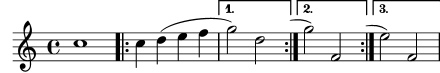#### Known issues and warnings

It is not possible to modify shapes of ties or slurs by changing the `control-points` property if there are multiple ties or slurs at the same musical moment – the `\tweak` command will also not work in this case. However, the `tie-configuration` property of `TieColumn` can be overridden to set start line and direction as required.